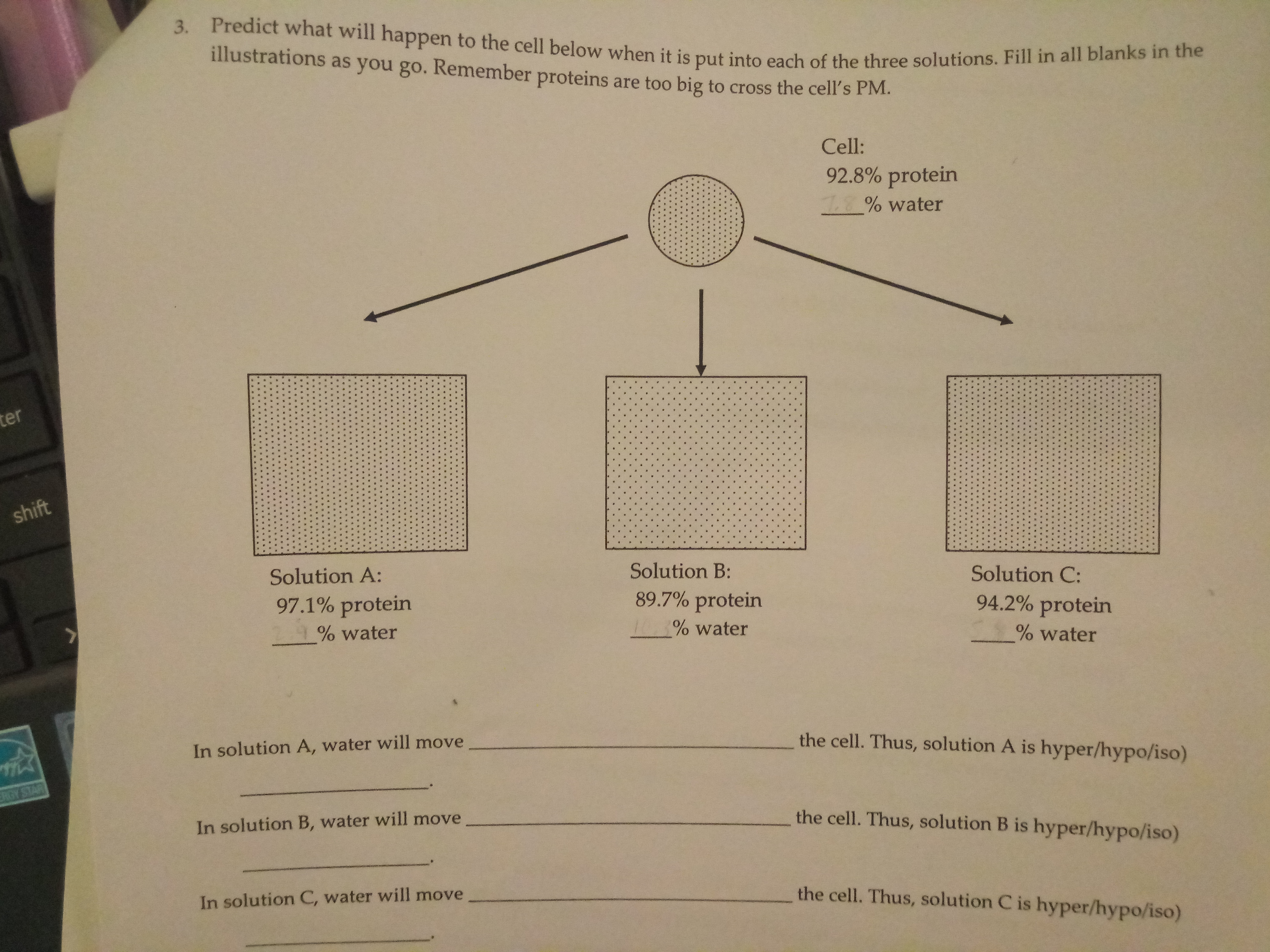# 3. Predict what will happen to the cell below when it is put into each of the three solutions. Fill in all blanks in the illustrations as you go. Remember proteins are too big to cross the cell's PM. Cell: 92.8% protein % water ter shift Solution A: Solution B: Solution C: 89.7% protein 97.1% protein 94.2% protein % water % water % water In solution A, water will move the cell. Thus, solution A is hyper/hypo/iso) ERGY STAR In solution B, water will move the cell. Thus, solution B is hyper/hypo/iso) In solution C, water will move the cell. Thus, solution C is hyper/hypo/iso)

Questionhelp_outlineImage Transcriptionclose3. Predict what will happen to the cell below when it is put into each of the three solutions. Fill in all blanks in the illustrations as you go. Remember proteins are too big to cross the cell's PM. Cell: 92.8% protein % water ter shift Solution A: Solution B: Solution C: 89.7% protein 97.1% protein 94.2% protein % water % water % water In solution A, water will move the cell. Thus, solution A is hyper/hypo/iso) ERGY STAR In solution B, water will move the cell. Thus, solution B is hyper/hypo/iso) In solution C, water will move the cell. Thus, solution C is hyper/hypo/iso) fullscreen